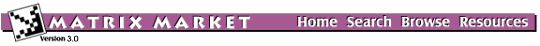## MATPDE: Partial Differential Equation

### from the NEP Collection

Matrix Generator MATPDE
Source: H. Elman, University of Maryland
Discipline: Partial differential equations
Language: Fortran
Output format: compressed row

This generator computes a five-point central finite difference discretization of the two-dimensional variable-coefficient linear elliptic equation -(p ux)x -(q uy)y + r ux + (r u)x + s uy + (s u)y + t u = f, where p, q, r, s and t are the functions of x and y. The domain is the unit square (0,1)x(0,1), and the boundary condition are Dirichlet.

Although the code handles arbitrary p, q, r, s and t, it is set up initially with p = exp(-xy), Q = exp(xy), R = beta (x+y), S = gamma (x+y), and t = 1/(1+x+y). It is suggested to use values of beta and gamma between 0 and 250. The object is to estimate those eigenvalues with the largest real parts and to determine whether or not there are significant gaps in the spectrum.

Parameters:

 NX number of grid points along x axis NY number of grid points along y axis BETA coefficient multiplying ux terms GAMMA coefficient multiplying uy terms

## Matrix Instances:

The Matrix Market is a service of the Mathematical and Computational Sciences Division / Information Technology Laboratory / National Institute of Standards and Technology.

[ Home ] [ Search ] [ Browse ] [ Resources ]

Last change in this page: Wed Sep 22 13:37:29 US/Eastern 2004 [Comments: ]